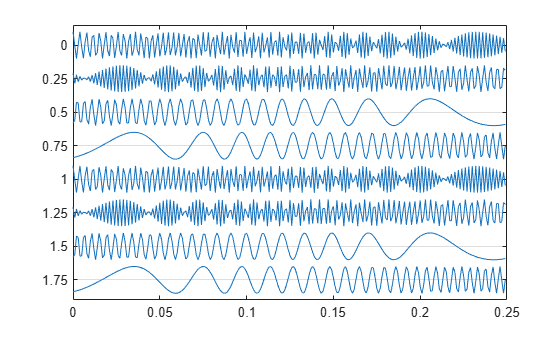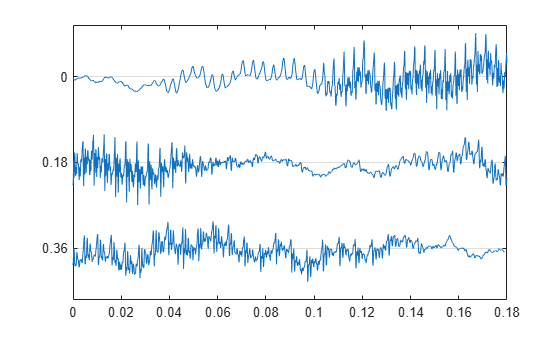# strips

## Syntax

```strips(x) strips(x,n) strips(x,sd,fs) strips(x,sd,fs,scale) ```

## Description

`strips(x)` plots vector `x` in horizontal strips of length 250. If `x` is a matrix, `strips(x)` plots each column of `x`. The left-most column (column 1) is the top horizontal strip.

`strips(x,n)` plots vector `x` in strips that are each `n` samples long.

`strips(x,sd,fs)` plots vector `x` in strips of duration `sd` seconds, given a sampling frequency of `fs` samples per second.

`strips(x,sd,fs,scale)` scales the vertical axes.

If `x` is a matrix, `strips(x,n)`, `strips(x,sd,fs)`, and `strips(x,sd,fs,scale)` plot the different columns of `x` on the same strip plot.

`strips` ignores the imaginary part of complex-valued `x`.

## Examples

collapse all

Plot two seconds of a frequency-modulated sinusoid in 0.25 second strips. Specify a sample rate of 1 kHz.

```fs = 1000; t = 0:1/fs:2; x = vco(sin(2*pi*t),[10 490],fs); strips(x,0.25,fs)```Load a speech signal sampled at ${F}_{s}=7418\phantom{\rule{0.2777777777777778em}{0ex}}Hz$. The file contains a recording of a female voice saying the word "MATLAB®."

`load mtlb`

Plot the signal in 0.18 second long strips.

`strips(mtlb,0.18,Fs)`